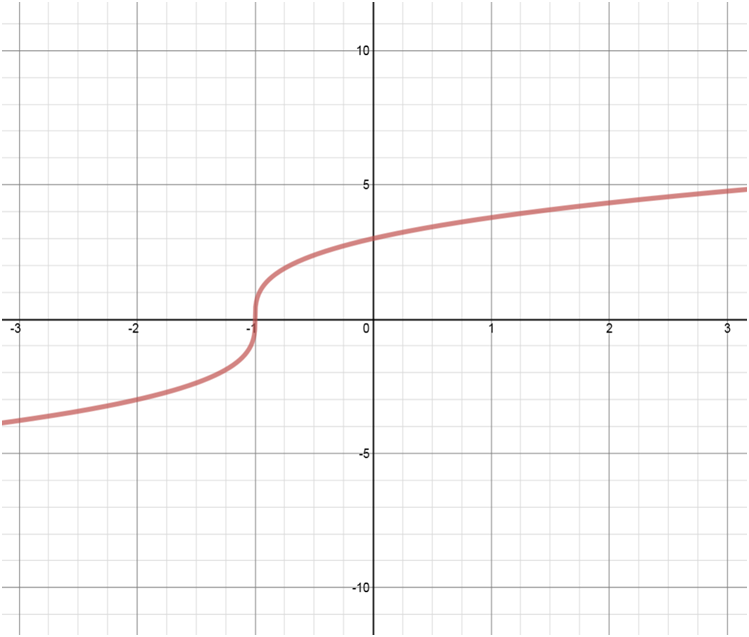# Transformations of functions: Vertical translations - Relations and Functions

### Transformations of functions: Vertical translations

Vertical translations refer to movements of a graph of a function vertically along the y-axis by changing the y values. So, if y = f(x), then y = (x) + h results in a vertical shift. If h > 0, then the graph shifts h units up; while If h < 0, then the graph shifts h units down.

#### Lessons

##### In conclusion: • $\left( y \right) \to \left( {y + 8} \right)$: shift ________ units ______________ $\Rightarrow$ all $y$ coordinates _____________________________. • $\left( y \right) \to \left( {y - 3} \right)$: shift ________ units ______________ $\Rightarrow$ all $y$ coordinates _____________________________.